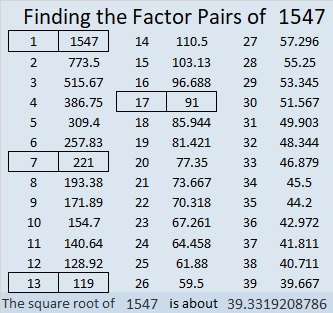# 1547 is a Hexagonal Pyramidal Number

Contents

### Today’s Puzzle:

1547 is the 13th hexagonal pyramidal number. Looking at the graphic that I made below of 1547 tiny squares, can you determine what the prime factorization of 1547 is?### Factors of 1547:

• 1547 is a composite number.
• Prime factorization: 1547 = 7 × 13 × 17.
• 1547 has no exponents greater than 1 in its prime factorization, so √1547 cannot be simplified.
• The exponents in the prime factorization are 1, 1, and 1. Adding one to each exponent and multiplying we get (1 + 1)(1 + 1)(1 + 1) = 2 × 2 × 2 = 8. Therefore 1547 has exactly 8 factors.
• The factors of 1547 are outlined with their factor pair partners in the graphic below.### More about the Number 1547:

1547 is the hypotenuse of FOUR Pythagorean triples:
147-1540-1547, which is 7 times (21-220-221),
595-1428-1547, which is (5-12-13) times 119,
728-1365-1547, which is (8-15-17) times 91, and
980-1197-1547, which is 7 times (140-171-221).

This site uses Akismet to reduce spam. Learn how your comment data is processed.